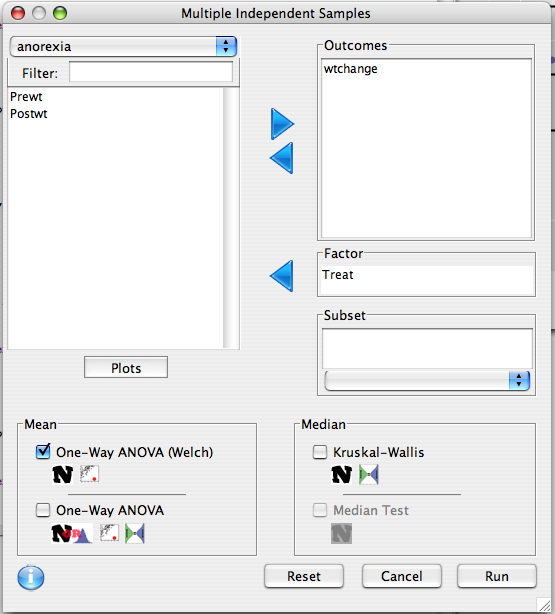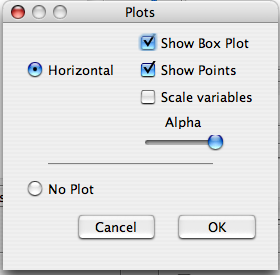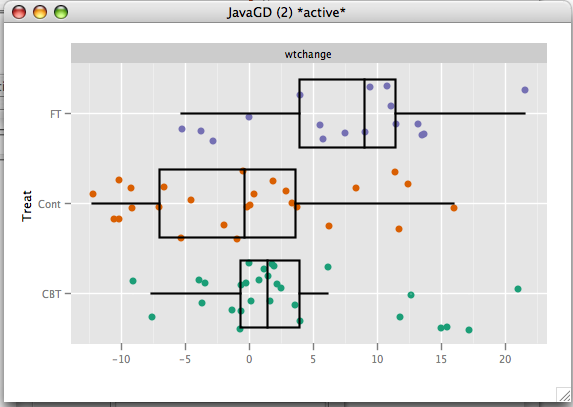Tests equality of distribution in two or more groups.

## Dialog

Variables in the outcome list should be continuous (or at least ordinal for the kruskal-wallis test). The factor variable should be categorical.The subset text feild allows one to specify that the test is to be done only in a subset of the data frame.

### Plots

The plot button gives box and jitter plots.## Tests

### Welch Anova

The Welch Anova, which is a generalization of the Welch t-test, tests whether the means of the outcome variables are different across the factor levels. It Assumes that the sample sizes are sufficiently large, and is sensitive to the existence of outliers.

### Anova

Similar to the Welch Anova, the classical Anova tests equality of means. Unlike the Welch test, it requires that the variances of the outcomes across the factor levels is the same, and is exact at small sample sizes if the distribution is exactly normal. Detail

### kruskall-Wallis

The Kruskall-Wallis procedure tests whether the medians of the distributions are the same. Unlike the Anova, this test is not sensitive to the presence of outliers. More information is available here.